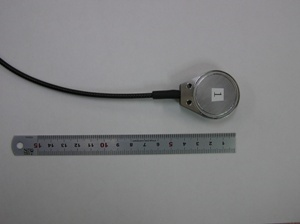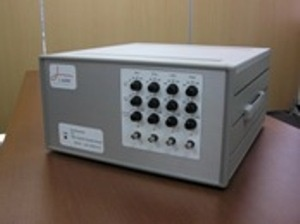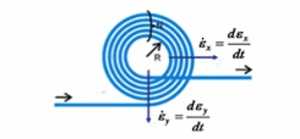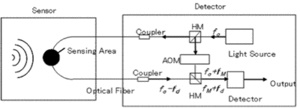This system can detect Acoustic Emission (AE) upto 1 MHz.

This is mainly utilized at :
– Underground tunnel for rock monitoring
– Crack detection
– Corrosion detection
– Rotating abnormality monitoring

>> FOD sensing system (application/pdf:542.1 KB)

■Technical Information

 Fiber Optical Doppler Sensor (FOD)

FOD sensor can detect ultrasonic wave (vibration at the same time) that cannot be heard by human ear. Conventionally, several kinds of ceramics are used, where FOD can compete. In material science, by hearing very small ultrasonic, material destruction process is observed from micro crack till further degradation.

A sensing region of the fiber optic is attached on a measured object as shown below. When the object vibrates with some elastic motion on surface, the attached fiber optic elongates and shortens simultaneously. If the light wave of frequency fo is emitted into a corresponding fiber optic region, change in length of fiber optic causes a laser frequency modulation, because the number of light waves in that region is constant in a moment. In other words, the frequency modulates by fd because the light velocity is constant. This is called “Laser Doppler effect” that the frequency of light wave transmitted from other part is given “fo – fd”. The frequency modulation fd is proportional to the velocity of fiber optical length, i.e., the displacement rate of attached region.

Frequency modulation by Doppler effect is described as formula below. fd as modulated frequency, λ as light wavelength, and dL/dt as displacement rate. The negative (-) indicates that the frequency modulation is opposite to displacement rate in terms of phase analysis (+),(-).

fd = -1/λ*dL/dt

As shown in the formula above, the frequency modulation fd and the displacement rate dL/dt are proportional. The frequency modulation fd is detected using the heterodyne interferometry.

Figure below shows the Laser Doppler Interferometry system. The laser with frequency fo is emitted and divided into the sensing optical path and detecting optical path. Where measured object has a certain displacement at sensing region dynamically, the length of optical path changes accordingly. Then the proportional frequency modulation fd occurs to the laser light, which is “fo – fd”. At detecting optical path, frequency fM (80 MHz) is added by AOM (Acousto Optical Modulator) to create the frequency “fo + fM”. The difference in frequency given as “fM + fd” is converted into the voltage.